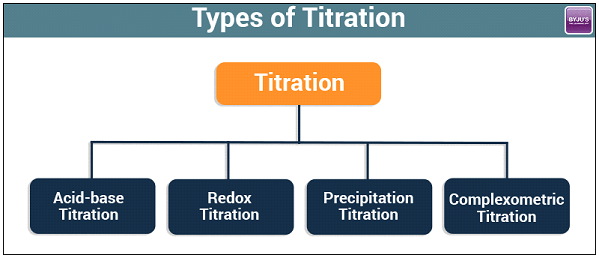# Types of Titration (Titration Chemistry)

## What is Titration?

A quantitative and volumetric technique, to determine the unknown concentration of a solution by the known concentration of a solution in the presence of indicator is called Titration

Titration is a common laboratory method of using quantitative chemical analysis. This method is used to determine the unidentified concentration of a known analyte. The volume measurement is known as volumetric analysis, and it is important in the titration.

## Types of Titration

There are many types of titration when considering goals and procedures. However, the most common types of titration in quantitative chemical analysis are redox titration and acid-base titration.

Titrations can be classified as:

1. Acid-base Titrations
2. Redox Titrations.
3. Precipitation Titrations.
4. Complexometric Titrations.Four Types of Titration

## Recommended Videos

### Titration using acidified KMnO4 and acidified K2Cr2O7## 1. Acid-Base Titration

The strength of an acid can be determined using a standard solution of a base. This process is called acidimetry. In the same way, the strength of a base can be found with the help of a standard solution of an acid, which is known as alkalimetry. Both titrations involve in the neutralization reaction of an alkali.

### What is Acid-Base Titration?

It is a quantitative analysis method to determine an acid’s or bases’ concentration by precisely neutralizing them with a standard solution of either acid or base of known concentration. It is monitored with the help of a pH indicator to know the development of the acid-base reaction.

HA+BOH→BA+H2O

Acid + Alkali→Salt + Water

Or H+ + A– + B+ + OH → B+ + A + H2O

Or H+ + OH → H2O

The acid-base titration is based on the reaction that neutralization is between a base or an acidic and analyte. In this type, a reagent is mixed with the sample solution until it reaches the required pH level. This type of titration majorly depends on the track change in pH or a pH meter.

## Recommended Videos

### Acid-Base Titrations## 2. Redox Titrations

The redox titration is also known as an oxidation-reduction reaction. In this type of titration, the chemical reaction takes place with a transfer of electrons in the reacting ions of aqueous solutions. The titrations are named after the reagent that is used in are as follows;

• Permanganate Titrations
• Dichromate Titrations
• Iodimetric and Iodometric Titrations

### Permanganate Titrations

In this titration, the potassium permanganate is used as an oxidizing agent. It is maintained with the use of dilute sulphuric acid. Here is the equation.

2KMnO4 + 3H2SO4 → K2SO4 + 2MnSO4 + 3H2O + 5[O]

Or MnO4 + 8H+ + 5e → Mn2++ 4H2O

Further, the solution remains colourless before the endpoint. The potassium permanganate is used to estimate oxalic acid, ferrous salts, hydrogen peroxide, oxalates and more. While the solution of potassium permanganate is always standardized before it is used.

### Dichromate Titrations

These are titrations in which, potassium dichromate is used as an oxidising agent in acidic medium. The medium is maintained acidic by the use of dilute sulphuric acid. The potential equation is:

K2Cr2O7 + 4H2SO4 K2SO4 + Cr2(SO4)3 + 4H2O + 3[O]

Or Cr2O72 + 14H+ + 6e → 2 Cr3+ + 7H2

The solution of potassium dichromate can be directly used for titrations. It is mainly used for the estimation of ferrous salts and iodides.

### Iodimetric and Iodometric Titrations

The reduction of free iodine to iodide ions and

oxidation of iodide ions to free occurs in these titrations.

l2 + 2e– → 2l……………. (reduction)

2l → I2  + 2e ……………. (oxidation)

The solution is used as an indicator. Free iodine is used in the iodometric titration, while in the iodometric titration an oxidation agent is used to react to liberate free iodine.

## 3. Precipitation Titrations

The titration is based on the insoluble precipitate formation when the two reacting substances are brought into contact are called precipitation titration. For instance, when the solution of silver nitrate is used to a solution of ammonium thiocyanate or sodium chloride, it reacts and forms a white precipitate of silver thiocyanate or silver chloride.

AgNO3 + NaCl  AgCl + NaNO3

AgNO3 + NH4CNS AgCNS + NH4NO3

## 4. Complexometric Titrations

The complexometric titration is where an undissociated complex is formed at an equivalence point. It is greater than the precipitation titrations, and there will be no error due to co-precipitations.

Hg2+ + 2SCN Hg(SCN)2

Ag+ + 2CN–  [Ag(CN)2]

Ethylenediaminetetraacetic acid (EDTA) is an important reagent that forms complexes with metals.

## Frequently Asked Questions – FAQs

### What is the main purpose of an acid-base titration?

To determine the unknown concentration of a base or acid by neutralizing them with a base or n acid of a known concentration.

### What are acid-base indicators?

Substances that change their colour when the pH of their surrounding changes are called acid-base indicators. They are also called pH indicators.

### What is the purpose of titration in chemistry?

Titrating a simple solution with a volume of a regular acid solution (of known concentration) needed to neutralize it may be used to determine its concentration. The aim of the titration is to find the equivalence point, which is the point where chemically equivalent quantities of the reactants are combined.

### What does it mean to titrate a solution?

Titration is the incremental addition of a known concentration solution (called a titrant) to a known volume of an unknown concentration solution before the reaction achieves neutralization, which is often signaled by a color shift.

### What is end point in titration?

When an indicator indicates that the amount of reactant needed for a full reaction has been added to a solution during a titration.

Test Your Knowledge On Types Of Titration!